# The Building Blocks of Matter Atoms Matter Anything

• Slides: 22The Building Blocks of Matter: Atoms - ++ + + + - -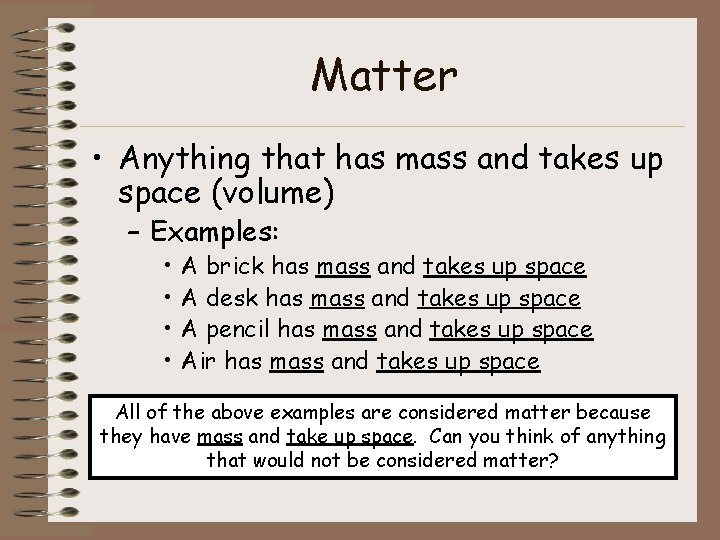Matter • Anything that has mass and takes up space (volume) – Examples: • • A brick has mass and takes up space A desk has mass and takes up space A pencil has mass and takes up space Air has mass and takes up space All of the above examples are considered matter because they have mass and take up space. Can you think of anything that would not be considered matter?Atoms • Smallest possible unit into which matter can be divided, while still maintaining its properties. - + + - - • Made up of: For example, what is the + – protons smallest possible unit which a long essay can be – into neutrons divided and still have some meaning? – electrons - • The solar system is commonly used as an analogy to describe the structure of an atom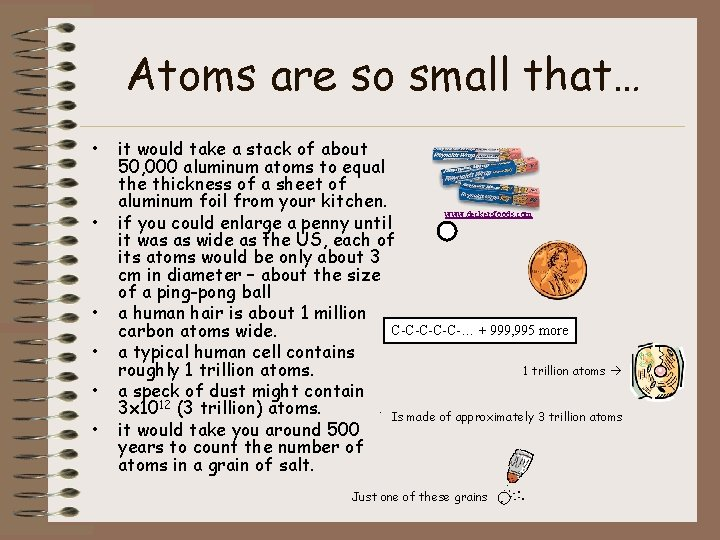Atoms are so small that… • • • it would take a stack of about 50, 000 aluminum atoms to equal the thickness of a sheet of aluminum foil from your kitchen. www. deckersfoods. com if you could enlarge a penny until it was as wide as the US, each of its atoms would be only about 3 cm in diameter – about the size of a ping-pong ball a human hair is about 1 million C-C-C-… + 999, 995 more carbon atoms wide. a typical human cell contains 1 trillion atoms roughly 1 trillion atoms. a speck of dust might contain. 3 x 1012 (3 trillion) atoms. Is made of approximately 3 trillion atoms it would take you around 500 years to count the number of atoms in a grain of salt. Just one of these grains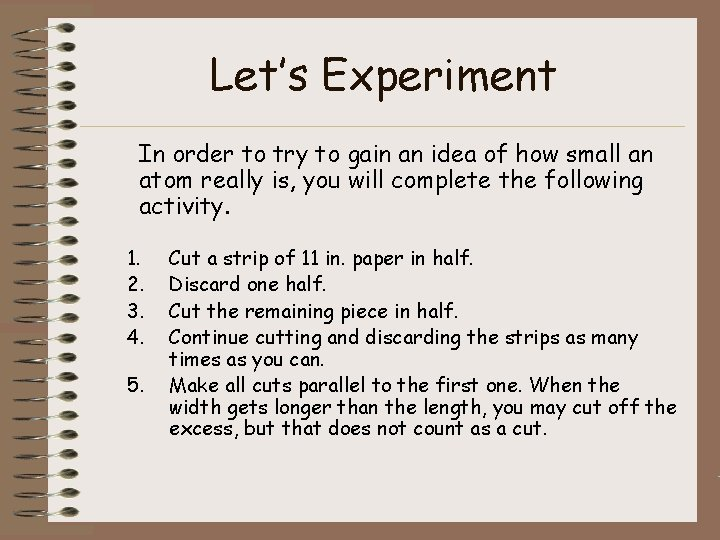Let’s Experiment In order to try to gain an idea of how small an atom really is, you will complete the following activity. 1. 2. 3. 4. 5. Cut a strip of 11 in. paper in half. Discard one half. Cut the remaining piece in half. Continue cutting and discarding the strips as many times as you can. Make all cuts parallel to the first one. When the width gets longer than the length, you may cut off the excess, but that does not count as a cut.Results • How many cuts were you able to make? • Do you think you could keep cutting the paper forever? Why or why not? You would have to cut the paper in half around thirty-one (31) times to get to the size of any atom. http: //www. miamisci. org/af/sln/phantom/papercutting. html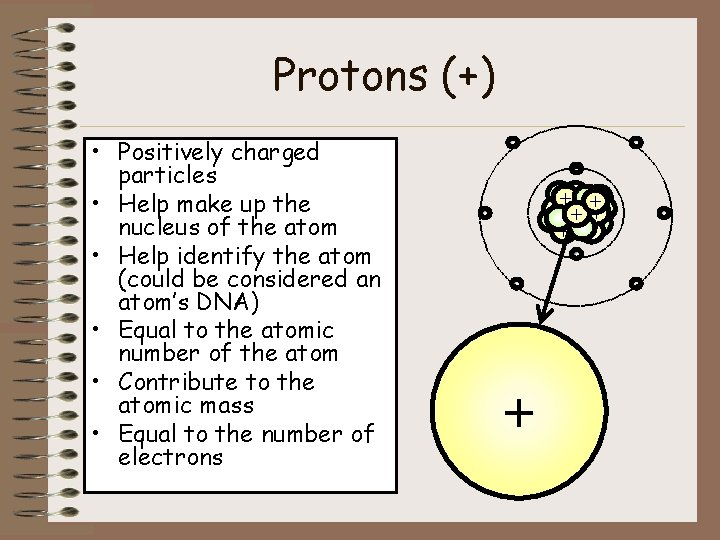Protons (+) • Positively charged particles • Help make up the nucleus of the atom • Help identify the atom (could be considered an atom’s DNA) • Equal to the atomic number of the atom • Contribute to the atomic mass • Equal to the number of electrons - ++ + + + - - + -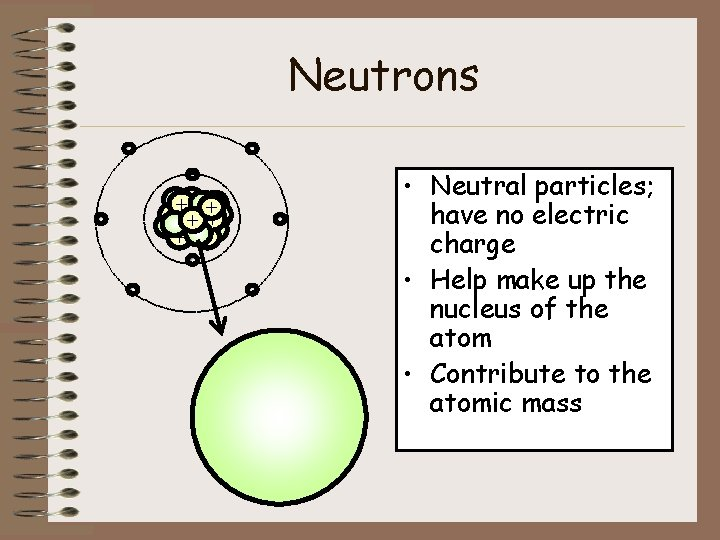Neutrons - ++ + + + - - - • Neutral particles; have no electric charge • Help make up the nucleus of the atom • Contribute to the atomic mass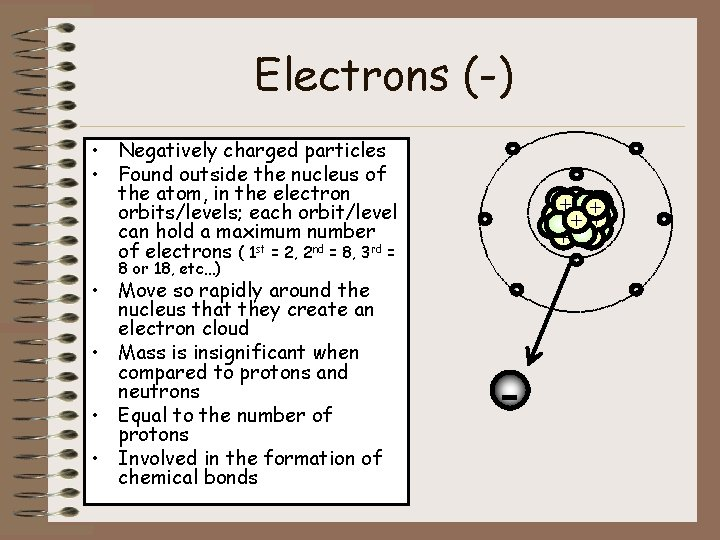Electrons (-) • Negatively charged particles • Found outside the nucleus of the atom, in the electron orbits/levels; each orbit/level can hold a maximum number of electrons ( 1 st = 2, 2 nd = 8, 3 rd = ++ + + + - - 8 or 18, etc…) • Move so rapidly around the nucleus that they create an electron cloud • Mass is insignificant when compared to protons and neutrons • Equal to the number of protons • Involved in the formation of chemical bonds - -The Atom’s “Center” • Protons and neutrons are grouped together to form the “center” or nucleus of an atom. Notice that the electrons are not apart of the nucleus - + + -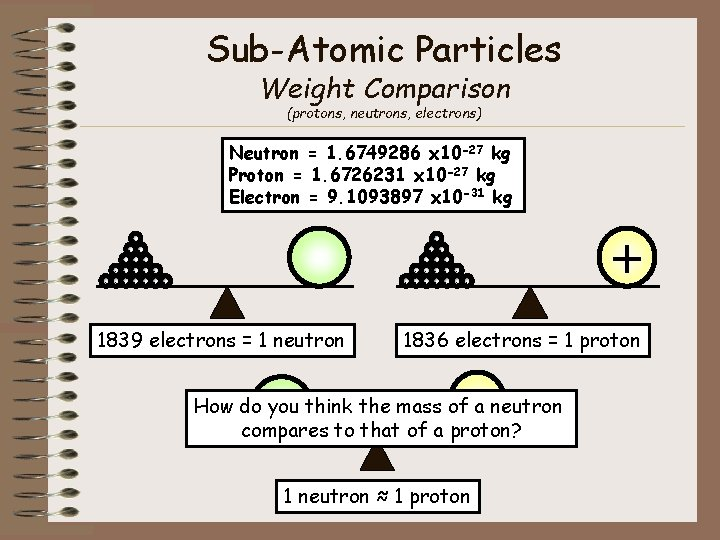Sub-Atomic Particles Weight Comparison (protons, neutrons, electrons) Neutron = 1. 6749286 x 10 -27 kg Proton = 1. 6726231 x 10 -27 kg Electron = 9. 1093897 x 10 -31 kg - - - - - - - 1839 electrons = 1 neutron + 1836 electrons = 1 proton + How do you think the mass of a neutron compares to that of a proton? 1 neutron ≈ 1 protonSub-atomic Particles Size Comparison (protons, neutrons, electrons, & quarks) Size in atoms - Size in meters (m) - Atom 1 10 -10 Nucleus __1__ 10, 000 10 -14 Proton or Neutron ___1___ 100, 000 10 -15 ++ + + + Electron or Quark _____1____ 100, 000 10 -18 - - - (at largest) - -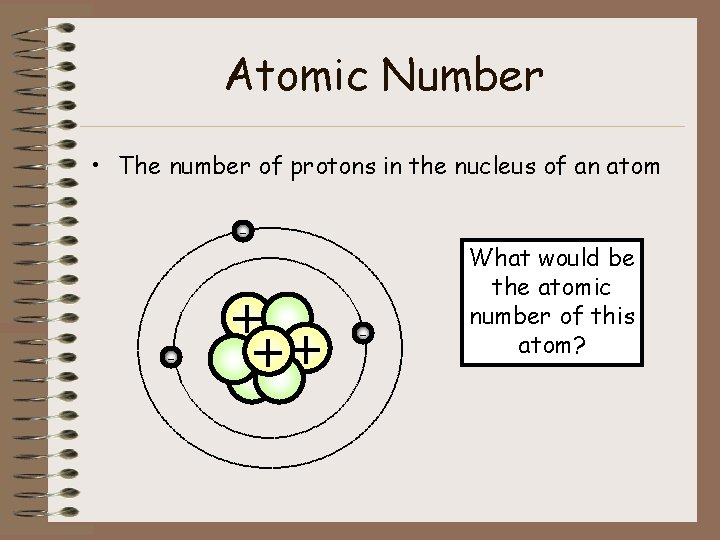Atomic Number • The number of protons in the nucleus of an atom - - + ++ - What would be the atomic number of this atom?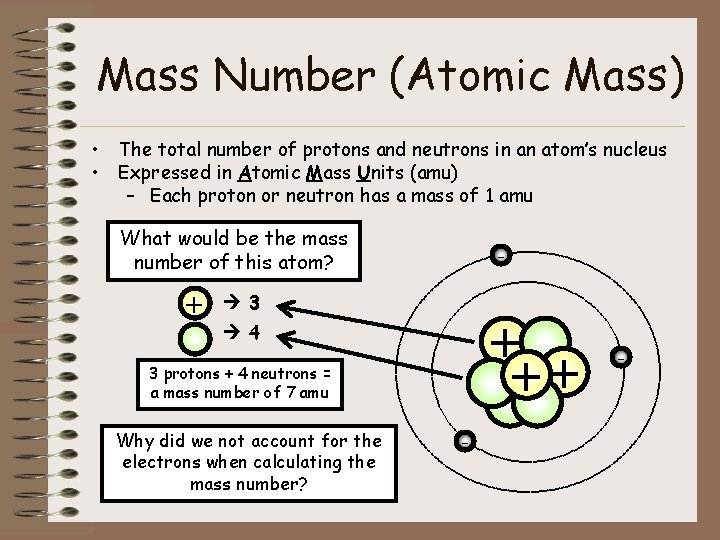Mass Number (Atomic Mass) • The total number of protons and neutrons in an atom’s nucleus • Expressed in Atomic Mass Units (amu) – Each proton or neutron has a mass of 1 amu What would be the mass number of this atom? + - 3 + ++ 4 3 protons + 4 neutrons = a mass number of 7 amu Why did we not account for the electrons when calculating the mass number? - -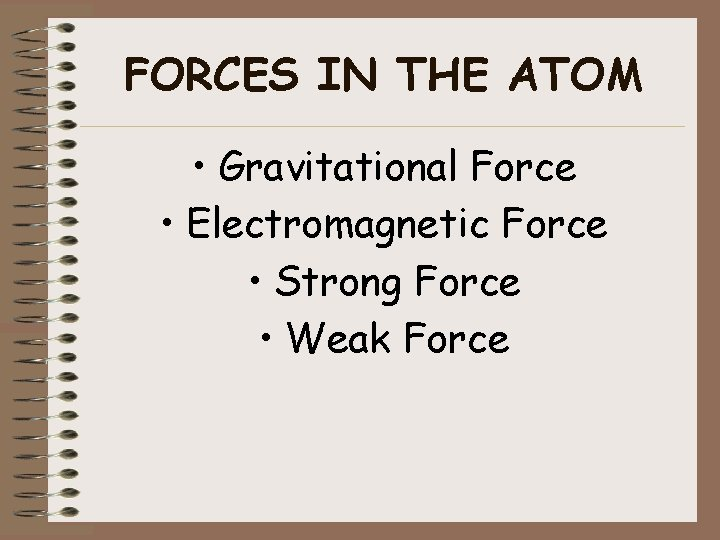FORCES IN THE ATOM • Gravitational Force • Electromagnetic Force • Strong Force • Weak ForceGravitational Force • The force of attraction of objects due to their masses • The amount of gravity between objects depends on their masses and the distance between them Do you think this force plays a significant role in holding the atom together?Electromagnetic Force • The force that results from the repulsion of like charges and the attraction of opposites • The force that holds the electrons around the nucleus Why are neutrons not pictured above? + + + - Notice how the particles with the same charge move apart and the particles with different charges move together.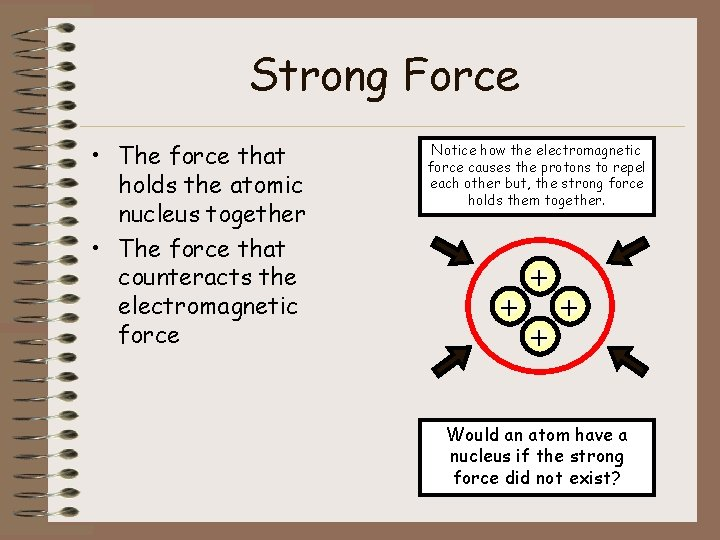Strong Force • The force that holds the atomic nucleus together • The force that counteracts the electromagnetic force Notice how the electromagnetic force causes the protons to repel each other but, the strong force holds them together. + + Would an atom have a nucleus if the strong force did not exist?Weak Force • This force plays a key role in the possible change of sub-atomic particles. – For example, a neutron can change into a proton(+) and an electron(-) Notice how the original particle changes to something new. n +- • The force responsible for radioactive decay. – Radioactive decay process in which the nucleus of a radioactive (unstable) atom releases nuclear radiation. If you need help remembering weak force, just think of…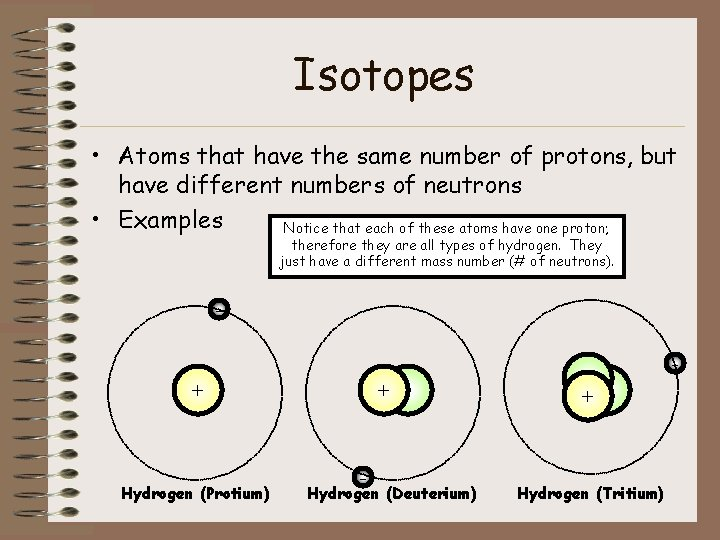Isotopes • Atoms that have the same number of protons, but have different numbers of neutrons • Examples Notice that each of these atoms have one proton; therefore they are all types of hydrogen. They just have a different mass number (# of neutrons). + Hydrogen (Protium) + - Hydrogen (Deuterium) + Hydrogen (Tritium)Average Atomic Mass • • The weighted average of the masses of all the naturally occurring isotopes of an element The average considers the percent abundance of each isotope in nature Found on the periodic table of elements Example What would be the atomic mass (≈) of Hydrogen if these three isotopes were found in the following percentages (99. 9, 0. 015, 0) respectively? + Hydrogen (Protium) Mass # = 1 amu + - Hydrogen (Deuterium) Mass # = 2 amu + Hydrogen (Tritium) Mass # = 3 amu If you simply average three, 2 amu (1 amu + 2 amu + 3 amu/3) would be the atomic mass, but since 99. 9% of the Hydrogen is Protium, the atomic mass is around 1 amu (. 999 x 1 amu) -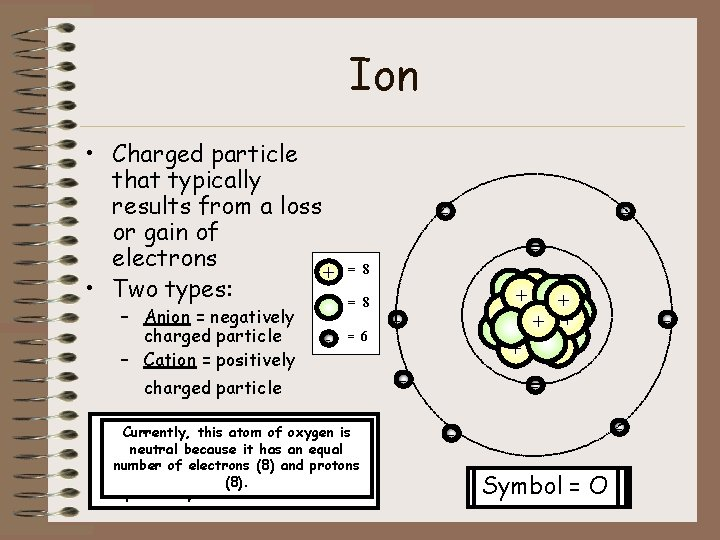Ion • Charged particle that typically results from a loss or gain of electrons + • Two types: – Anion = negatively charged particle – Cation = positively - - - = 8 = 968 charged particle Now that three this atom electrons ofof oxygen were just lost, Currently, this atom oxygen is gained the number an electron, of electrons it is no (6) longer and neutral because it has an equal protons neutral (8) an is atom. still (8) unbalanced; It is protons now number of or electrons and considered therefore, an it is ionstill (anion). but ion nowhas it (8). an ion, This ismore specifically electrons referred (9) thantoprotons as a cation. (8). - ++ + + + - - - 2+ Symbol == O O 1 -